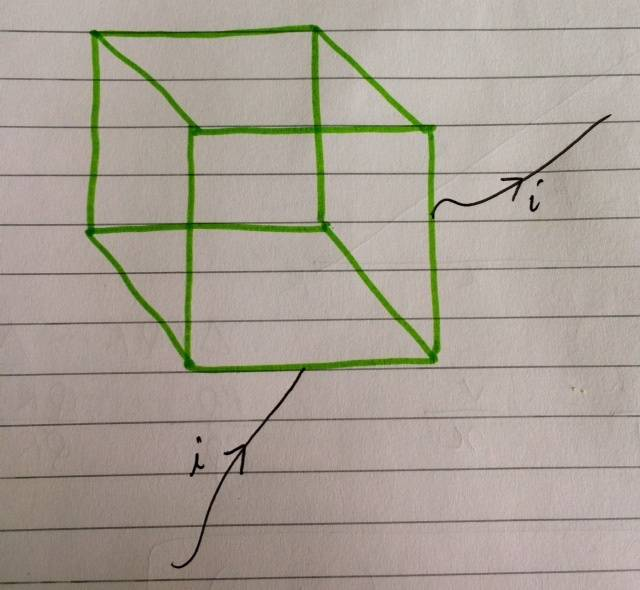# Magnetic field - General current in a cube

pieuler
Homework Statement:
Given a cube carrying current, such that the current enters and leaves the cube at any two arbitrary points, prove that the magnetic field at the centre of the cube will always be 0.
Relevant Equations:
Biot Savart Law
Ampere Circuit law
I could solve a similar (rather, a specific case of the above) where the current entered through a
corner and left from the corner opposite to it along the body diagonal of the cube. For this specific case, I was able to easily exploit symmetry to deduce the answer (0). However, I cannot think of any suitable technique (eg. symmetry) for the general version.

PS. I have basic knowledge of calculus and vectors, but not so much of 'vector calculus'. Any intuitive guidance/hints/solutions are appreciated.

Last edited:

Homework Helper
Gold Member
I think we need to know more information about the given current density. Saying that it just enters at one point and exits at another point is not enough. We need to know what is the in between path (is it a straight line? it is some sort of helix? or what else, they can be infinite ways for the inbetween path) and how the current density varies spatially in this path.

If you have some image or figure you can post here please do it, many times one picture worth thousands of words.

I don't think we can prove that the magnetic field at the center will always be zero, regardless of the details of the current density.

pieuler
Current is constant and uniform. No specific details about current/current density were given so I believe it's supposed to be constant and uniform(like no variations whatsoever). Current flows along the edges only.Basically, any two completely random points on the edges of the cube. The setup is like a cubical cage made of wires. So that tells you that the current flows only along the edges.

•Delta2
Homework Helper
Gold Member
I still don't see how it would be possible to prove that the magnetic field would be zero at the center, my opinion is that it depends on the entry point, on the exit point and which edges (or parts of edges) are "active" that is they carry the current.

pieuler
I still don't see how it would be possible to prove that the magnetic field would be zero at the center, my opinion is that it depends on the entry point, on the exit point and which edges (or parts of edges) are "active" that is they carry the current.
How about we consider the edges to be resistances (equal of course) and then think about the current distribution? That is one way of doing the specific case I mentioned, so it might help in the general case too.